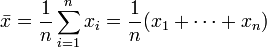Mar
30

## Calculate average using AWK

The average or arithmetic mean is given asAssume we have an input file foo with f.ex. line number in first column and in the second column (\$2 in awk) we have the values of interest.

File: foo

1 2
2 3
3 6
4 8
5 11

Use the following awk commando to calculate average or arithmetic mean

awk ‘{s+=\$2} END{print “Sum: “s, “\nNumber of lines: “NR, “\nAverage: “s/(NR)}’ foo

The result will be:

Sum: 30
Number of lines: 5
Average: 6.0

#### Standard deviation

Here you may see how to calculate the standard deviation using AWK.

#### Minimum and maximum

Here you may find how to calculate the minimum and maximum values using AWK.

#### 1 comment

1.##### Isabelle Swartwout says:

Thanks for helping out, good information.